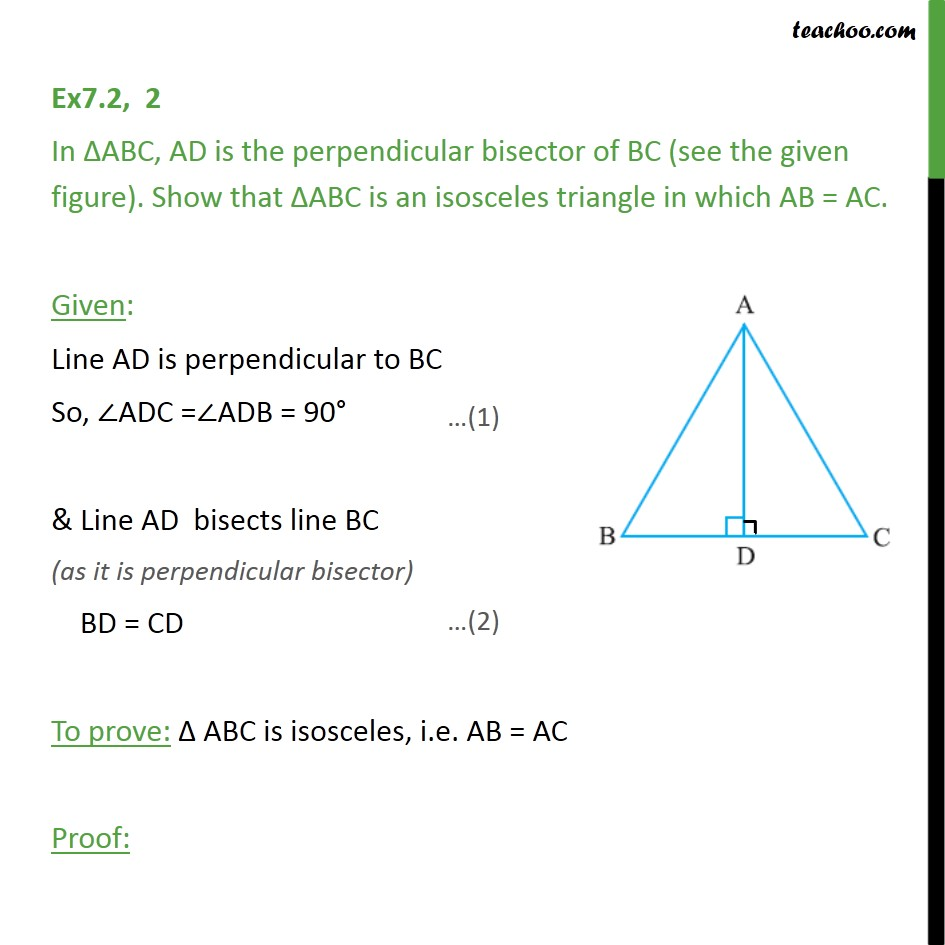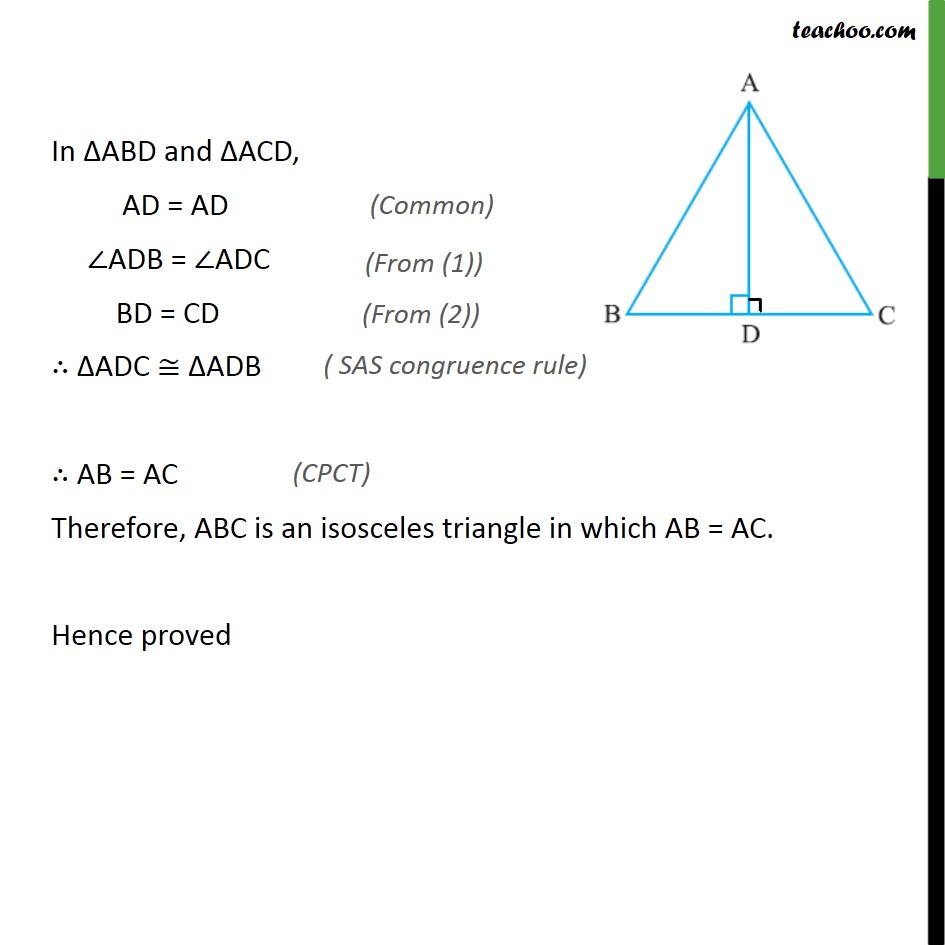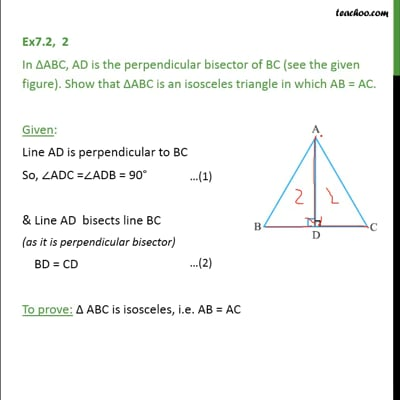Ex 7.2

Chapter 7 Class 9 Triangles
Serial order wiseThis video is only available for Teachoo black users

Solve all your doubts with Teachoo Black (new monthly pack available now!)

### Transcript

Ex7.2, 2 In ABC, AD is the perpendicular bisector of BC (see the given figure). Show that ABC is an isosceles triangle in which AB = AC. Given: Line AD is perpendicular to BC So, ADC = ADB = 90 & Line AD bisects line BC (as it is perpendicular bisector) BD = CD To prove: ABC is isosceles, i.e. AB = AC Proof: In ABD and ACD, AD = AD ADB = ADC BD = CD ADC ADB AB = AC Therefore, ABC is an isosceles triangle in which AB = AC. Hence proved